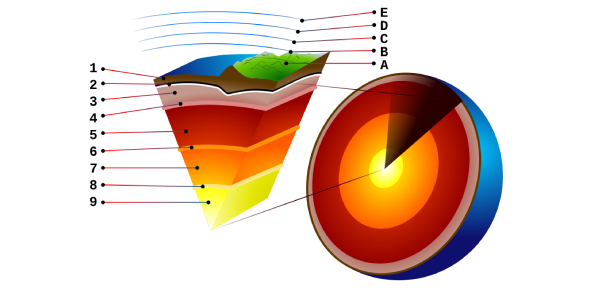# Quiz: Earth And Geology

100 Questions | Total Attempts: 348SettingsCreate your own Quiz.

• 1.
Which of the following features only forms underwater?
• A.

Aa

• B.

Pyroclasts

• C.

Pahoehoe

• D.

Pillow basalt

• E.

Volcanic breccia

• 2.
Ductile rocks deform by faulting
• A.

True

• B.

False

• 3.
What types of plate boundaries can be found along the western coast of the US
• A.

O-O divergent

• B.

C-C divergent

• C.

O-O convergent

• D.

O-C convergent

• E.

C-C convergent

• F.

O-O transform

• G.

C-C transform

• 4.
Where would you expect to find earthquakes and volcanism occurring together?
• A.

O-O divergent

• B.

C-C divergent

• C.

O-O convergent

• D.

O-C convergent

• E.

C-C convergent

• F.

O-O transform

• G.

C-C transform

• 5.
Where would you expect to find only earthquakes?
• A.

O-O divergent

• B.

C-C divergent

• C.

O-O convergent

• D.

O-C convergent

• E.

C-C convergent

• F.

O-O transform

• G.

C-C transform

• 6.
What type of volcano has alternating layers of lava and pyroclastic materials
• A.

Shield volcano

• B.

Cinder cone

• C.

Stratovolcano

• D.

Volcanic dome

• 7.
A (an) ____ eruption is characterized by lava flows through long, nearly vertical cracks in Earth's surface.
• A.

Fissure

• B.

Pyroclastic

• C.

Rhyolitic

• D.

Andesitic basaltic

• 8.
Calderas are larger features than craters
• A.

True

• B.

False

• 9.
Phenocrysts are solidified fragments of volcanic material that are ejected into the air
• A.

True

• B.

False

• 10.
Fumaroles are hot water fountains that spout intermittently
• A.

True

• B.

False

• 11.
Which of the following settings is LEAST likely to erupt basaltic lavas
• A.

Oceanic plate subducting underneath oceanic plate

• B.

Oceanic plate diverging from oceanic plate

• C.

Hot spot volcanism

• D.

Oceanic plate subducting underneath continental plate

• 12.
A (an) ___ is a torrential flow of wet  volcanic debris
• A.

Flank collapse

• B.

Eruption cloud

• C.

Caldera collapse

• D.

Earthquake

• E.

Lahar

• 13.
Which of the following items causes the most volcanic related fatalities
• A.

Tsunamis

• B.

Lahars

• C.

Pyroclastic flows

• D.

Ash

• E.

Floods

• 14.
Volcanically active areas can be good sources of heat energy for geothermal power
• A.

True

• B.

False

• 15.
If all the rocks in a mountain are basalt where would this mountain be
• A.

An ash flow deposit area

• B.

An area with a recent pyroclastic eruption

• C.

An area next to a caldera

• D.

An area near an active geyser field

• E.

A large igneous province area

• 16.
A granite formed with 1000 atoms of uranium-238 (a radioactive isotope). The rock now contains 500 atoms of uranium-237. How many half lives of uranium-238 have passed since the rock formed
• A.

0

• B.

1

• C.

2

• D.

3

• E.

4

• 17.
The relative age of the granite can be calculated knowing the half life of uranium-238 is 4.5 billion years
• A.

True

• B.

False

• 18.
In which eon did humans evolve
• A.

Archean

• B.

• C.

Phanerozoic

• D.

Proterozoic

• 19.
All eons encompass different amounts of geologic time
• A.

True

• B.

False

• 20.
You have identified an erosional surface located between gneiss (below the surface) and limestone (above the surface). What name BEST describes the erosional surface?
• A.

Unconformity

• B.

Disconformity

• C.

Angular unconformity

• D.

Nonconformity

• 21.
You have identified a basaltic dike in horizontal layers of a regionally extensive sandstone. what statement best describes the relationship between the dike and the sandstone?
• A.

The dike is younger than the sandstone

• B.

The sandstone is younger than the dike

• C.

The sandstone and the dike are the same age

• 22.
What statement best describes the principle of superposition
• A.

Sediments are deposited as essentially horizontal layers

• B.

Inclusions are older than the rocks they are contained in

• C.

Sediments spread out in all directions when they are deposited

• D.

Undisturbed sedimentary layers get younger from bottom to top

• 23.
Which of the following represents the smallest division of geologic time
• A.

Eon

• B.

Epoch

• C.

Era

• D.

Period

• 24.
A mass extinction is a short period in time in which a small percentage of species dies
• A.

True

• B.

False

• 25.
Which of the following can change the rate of radioactive decay
• A.

Changes in temperature

• B.

Chemical reactions

• C.

Changes in pressure

• D.

Nothing changes decay rates

Related TopicsBack to top
×

Wait!
Here's an interesting quiz for you.